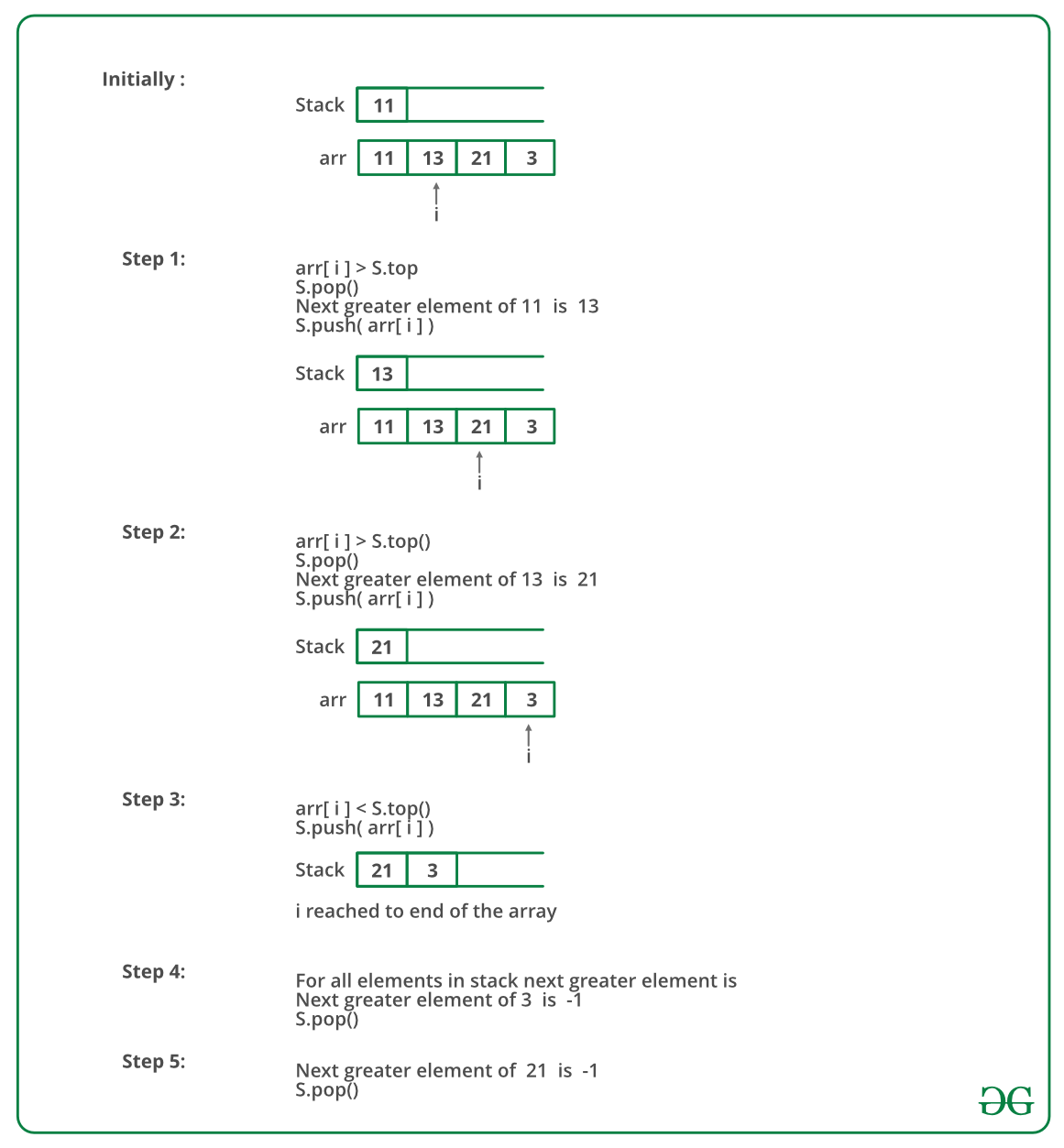# Java Program To Find Next Greater Element

• Last Updated : 17 Dec, 2021

Given an array, print the Next Greater Element (NGE) for every element. The Next greater Element for an element x is the first greater element on the right side of x in the array. Elements for which no greater element exist, consider the next greater element as -1.

Examples:

1. For an array, the rightmost element always has the next greater element as -1.
2. For an array that is sorted in decreasing order, all elements have the next greater element as -1.
3. For the input array [4, 5, 2, 25], the next greater elements for each element are as follows.
```Element       NGE
4      -->   5
5      -->   25
2      -->   25
25     -->   -1```

d) For the input array [13, 7, 6, 12}, the next greater elements for each element are as follows.

```  Element        NGE
13      -->    -1
7       -->     12
6       -->     12
12      -->     -1```

Method 1 (Simple)
Use two loops: The outer loop picks all the elements one by one. The inner loop looks for the first greater element for the element picked by the outer loop. If a greater element is found then that element is printed as next, otherwise, -1 is printed.

Below is the implementation of the above approach:

## Java

 `// Simple Java program to print next ``// greater elements in a given array`` ` `class` `Main``{ ``    ``/* prints element and NGE pair for ``     ``all elements of arr[] of size n */``    ``static` `void` `printNGE(``int` `arr[], ``int` `n)``    ``{``        ``int` `next, i, j;``        ``for` `(i=``0``; i

Output

```11 -- 13
13 -- 21
21 -- -1
3 -- -1```

Time Complexity: O(N2
Auxiliary Space: O(1)

Method 2 (Using Stack)

• Push the first element to stack.
• Pick rest of the elements one by one and follow the following steps in loop.
1. Mark the current element as next.
2. If stack is not empty, compare top element of stack with next.
3. If next is greater than the top element, Pop element from stack. next is the next greater element for the popped element.
4. Keep popping from the stack while the popped element is smaller than next. next becomes the next greater element for all such popped elements.
• Finally, push the next in the stack.
• After the loop in step 2 is over, pop all the elements from the stack and print -1 as the next element for them.

Below image is a dry run of the above approach:Below is the implementation of the above approach:

## Java

 `// Java program to print next``// greater element using stack`` ` `public` `class` `NGE {``    ``static` `class` `stack {``        ``int` `top;``        ``int` `items[] = ``new` `int``[``100``];`` ` `        ``// Stack functions to be used by printNGE``        ``void` `push(``int` `x)``        ``{``            ``if` `(top == ``99``) ``            ``{``                ``System.out.println(``"Stack full"``);``            ``}``            ``else``            ``{``                ``items[++top] = x;``            ``}``        ``}`` ` `        ``int` `pop()``        ``{``            ``if` `(top == -``1``) ``            ``{``                ``System.out.println(``"Underflow error"``);``                ``return` `-``1``;``            ``}``            ``else` `{``                ``int` `element = items[top];``                ``top--;``                ``return` `element;``            ``}``        ``}`` ` `        ``boolean` `isEmpty()``        ``{``            ``return` `(top == -``1``) ? ``true` `: ``false``;``        ``}``    ``}`` ` `    ``/* prints element and NGE pair for``       ``all elements of arr[] of size n */``    ``static` `void` `printNGE(``int` `arr[], ``int` `n)``    ``{``        ``int` `i = ``0``;``        ``stack s = ``new` `stack();``        ``s.top = -``1``;``        ``int` `element, next;`` ` `        ``/* push the first element to stack */``        ``s.push(arr[``0``]);`` ` `        ``// iterate for rest of the elements``        ``for` `(i = ``1``; i < n; i++) ``        ``{``            ``next = arr[i];`` ` `            ``if` `(s.isEmpty() == ``false``)``            ``{`` ` `                ``// if stack is not empty, then``                ``// pop an element from stack``                ``element = s.pop();`` ` `                ``/* If the popped element is smaller than``                   ``next, then a) print the pair b) keep``                   ``popping while elements are smaller and``                   ``stack is not empty */``                ``while` `(element < next) ``                ``{``                    ``System.out.println(element + ``" --> "``                                       ``+ next);``                    ``if` `(s.isEmpty() == ``true``)``                        ``break``;``                    ``element = s.pop();``                ``}`` ` `                ``/* If element is greater than next, then``                   ``push the element back */``                ``if` `(element > next)``                    ``s.push(element);``            ``}`` ` `            ``/* push next to stack so that we can find next``               ``greater for it */``            ``s.push(next);``        ``}`` ` `        ``/* After iterating over the loop, the remaining``           ``elements in stack do not have the next greater``           ``element, so print -1 for them */``        ``while` `(s.isEmpty() == ``false``)``        ``{``            ``element = s.pop();``            ``next = -``1``;``            ``System.out.println(element + ``" -- "` `+ next);``        ``}``    ``}`` ` `    ``// Driver Code``    ``public` `static` `void` `main(String[] args)``    ``{``        ``int` `arr[] = { ``11``, ``13``, ``21``, ``3` `};``        ``int` `n = arr.length;``        ``printNGE(arr, n);``    ``}``}`` ` `// Thanks to Rishabh Mahrsee for contributing this code`

Output

```11 --> 13
13 --> 21
3 --> -1
21 --> -1```

Time Complexity: O(N)
Auxiliary Space: O(N)

The worst case occurs when all elements are sorted in decreasing order. If elements are sorted in decreasing order, then every element is processed at most 4 times.

1. Initially pushed to the stack.
2. Popped from the stack when next element is being processed.
3. Pushed back to the stack because the next element is smaller.
4. Popped from the stack in step 3 of the algorithm.

How to get elements in the same order as input?

The above approach may not produce output elements in the same order as the input. To achieve the same order, we can traverse the same in reverse order

Below is the implementation of the above approach:

## Java

 `// A Stack based Java program to find next``// greater element for all array elements``// in same order as input.``import` `java.util.Stack;`` ` `class` `NextGreaterElement {`` ` `    ``static` `int` `arr[] = { ``11``, ``13``, ``21``, ``3` `};`` ` `    ``/* prints element and NGE pair for all``    ``elements of arr[] of size n */``    ``public` `static` `void` `printNGE()``    ``{``        ``Stack s = ``new` `Stack<>();``        ``int` `nge[] = ``new` `int``[arr.length];`` ` `        ``// iterate for rest of the elements``        ``for` `(``int` `i = arr.length - ``1``; i >= ``0``; i--)``        ``{``            ``/* if stack is not empty, then``            ``pop an element from stack.``            ``If the popped element is smaller``            ``than next, then``            ``a) print the pair``            ``b) keep popping while elements are``            ``smaller and stack is not empty */``            ``if` `(!s.empty())``            ``{``                ``while` `(!s.empty() ``                       ``&& s.peek() <= arr[i])``                ``{``                    ``s.pop();``                ``}``            ``}``            ``nge[i] = s.empty() ? -``1` `: s.peek();``            ``s.push(arr[i]);``        ``}``        ``for` `(``int` `i = ``0``; i < arr.length; i++)``            ``System.out.println(arr[i] + ``                               ``" --> "` `+ nge[i]);``    ``}`` ` `    ``/* Driver Code */``    ``public` `static` `void` `main(String[] args)``    ``{``        ``// NextGreaterElement nge = new``        ``// NextGreaterElement();``        ``printNGE();``    ``}``}`

Output

```11 ---> 13
13 ---> 21
21 ---> -1
3 ---> -1```

Time Complexity: O(N)
Auxiliary Space: O(N)

Please refer complete article on Next Greater Element for more details!

My Personal Notes arrow_drop_up